# Natural Convection and Entropy Generation of Nanofluids in A Square Cavity

Natural Convection and Entropy Generation of Nanofluids in A Square Cavity

Abd el malik BouchouchaRachid Bessaïh

LEAP Laboratory, Department of Mechanical Engineering, Faculty of Sciences Technology, University Frères Mentouri-Constantine, Route de Ain El. Bey, Constantine 25000, Algeria

Corresponding Author Email:
bouchoucha.malik@gmail.com
Page:
1-10
|
DOI:
http://dx.doi.org/10.18280/ijht.330401
|
Accepted:
|
Published:
31 December 2015
| Citation

OPEN ACCESS

Abstract:

The present paper investigates the natural convection and entropy generation of nanofluids in a square cavity. The left and right vertical walls of the cavity are maintained at a local high temperature TH (heat source) and a local cold temperature TC, respectively. The horizontal walls are adiabatic. The governing equations are discretised by using the finite volume method. A computer program based on the SIMPLER algorithm is developed and compared with the numerical results found in the literature. Results are presented in terms of the average Nusselt number and total entropy generation for a wide range of the Rayleigh number (104≤Ra≤106), solid volume fraction (0≤ϕ≤0.1) of nanoparticles, dimensionless heat source lengths (0.50≤B≤1), and different type of nanofluids. It is observed that the average Nusselt number ratio increases with the solid volume fraction, and the maximum total entropy generation ratio occurs at a low Rayleigh number for different values of ϕ.

Keywords:

Natural convection, Nanofluids, Cavity, Entropy generation.

1. Introduction

Nano-scale particle added fluids are called as a nanofluid; Choi  introduced this technique. Compared with the suspended particles of millimeters or higher, nanoﬂuids have greater stability and rheological properties, higher thermal conductivity and negligible pressure drop. Nanofluids seem to constitute a very interesting alternative for electronic cooling applications, micro-electromechanical systems, process heating/cooling to energy conversion and supply and magnet cooling, etc.

During the several past years, numerical studies of nanoﬂuid free convection in a square cavity were well studied and discussed [2-6]. In particular, Aminossadati and Ghasemi  investigated free convection of nanoﬂuid in a square cavity cooled from two vertical and horizontal walls and heated by a constant heat ﬂux on its horizontal bottom wall. It was found that type of nanoparticles and the length and location of the heat source affected signiﬁcantly the heat source maximum temperature. The same authors studied natural convection in an isosceles triangular enclosure with a heat source located at its bottom wall . Khanafer et al.  investigated the heat transfer enhancement in a two-dimensional enclosure using nanoﬂuids for various pertinent parameters. They tested different models for nanoﬂuid density, viscosity, and thermal expansion coefficients. It was found that the suspended nanoparticles substantially increase the heat transfer rate at any given Grashof number. Parvin et al. studied heat transfer and entropy generation through nanofluid filled direct absorption solar collector and the results revealed that both the mean Nusselt number and entropy generation increase as the volume fraction of Cu nanoparticles. Cheng [11-13] studied a diversity of free convection such as non-Newtonian nanofluids about a vertical

truncated cone in a porous medium, where he illustrated that increasing the thermophoresis parameter or the Brownian motion parameter tends to reduce the Nusselt number. Singh et al.  provided a study about entropy generation due to flow and heat transfer in nanofluids. They found that with the presence of a given nanofluid there is an optimum diameter at which entropy generation is minimum when both laminar and turbulent flow. Keblinski et al.  found that the increase of nanoﬂuids thermal conductivity is due to the Brownian motion of particles, the molecular-level layering of the liquid at the liquid/particle interface, the nature of heat transport in the nanoparticles, and the effect of nanoparticle clustering. Saleh et al.  numerically investigated heat transfer enhancement of nanoﬂuid in a trapezoidal enclosure using water–Cu and water–Al2O3, and developed a correlation of the average Nusselt number as a function of the angle of the sloping wall, effective thermal conductivity and viscosity as well as Grashof number. Jou and Tzeng  used nanoﬂuids to enhance natural convection heat transfer in a two-dimensional rectangular enclosure for various pertinent parameters. They conducted a numerical study using Khanafer’s model. They indicated that the solid volume fraction of nanoﬂuids causes an increase in the average heat transfer coefﬁcient. Oztop et al.  numerically analysed the problem of steady state natural convection in an enclosure filled with a nanofluid by using heating and cooling sinusoidal temperature profiles on one side. They found that the addition of nanoparticles into water affects the fluid flow and temperature distribution, especially for higher Rayleigh numbers. Hayat et al.  carried out an investigation on the flow of Power-Law Nanofluid over a stretching surface with Newtonian Heating. The result obtained have shown that Newtonian heating parameter and the volume fraction increases the thermal boundary layer thickness. On the other side, regarding the researches based on entropy generation.

Natural convection heat transfer of water-based nanoﬂuids in an inclined square enclosure has a very interesting case of study in the literature. The researchers investigated the effects of the inclination angle of the cavity, solid volume fractions, length of the constant heat ﬂux heater [20-21]. Mahmoodia and Hashemi  studied natural convection of a nanofluid in C-shaped enclosures. The discussed results show that where this last becomes narrower, the heat transfer rate increases. Besides the increasing of the Rayleigh number, the rate of heat transfer increases for a constant AR. Shahi et al.  studied the entropy generation due to natural convection cooling of a nanofluid; this last is consisted of water and Cu in protruded heat source cavity. Thus, they found that there is an inverse proportion between the Nusselt number and entropy generation.

In the present work, the objective is to investigate the steady laminar natural convection in a square cavity filled with nanofluid. The influence of the Rayleigh number, solid volume fraction, type of nanofluids and heat source length  on the average Nusselt number ratio and total entropy generation ratio were studied. This paper is organized as follows. Section 2 presents the geometry and mathematical model. Section 3 discusses the numerical method and code validation. Section 4 presents the results and discussion. Finally, a conclusion is given.

2. Mathematical Formulation

2.1 Problem description

Configurations considered in this study are shown in figures 1a-c. Each square cavity of dimension L is filled with pure water and nanofluid. The left vertical wall is kept at a local hot temperature TH, the right vertical wall is maintained at a local cold temperature TC, and the remaining boundaries are adiabatic. The base fluid (water) used is incompressible, Newtonian fluid that satisfies the Boussinesq hypothesis, and the nanofluids assumed to be incompressible and the flow is laminar. The thermo-physical properties of the nanofluid are constant, except for the variation of density, which is estimated by the Boussinesq hypothesis. The base fluid and spherical solid nanoparticles (Cu, Ag, Al2O3 and TiO2) are in thermal balance.

## 2a.png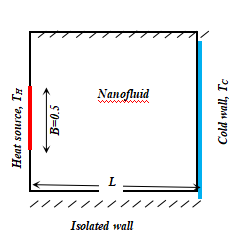(a) B=0.5

## 2b.png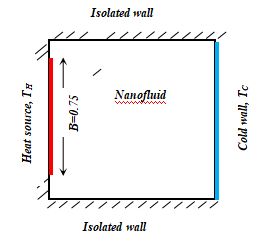(b) B=0.75

## 2c.png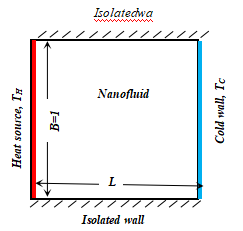(c) B=1

Figure 1. Geometeries and boundary conditions. L is the length of the cavity and B (normalized by L) is the dimensionless heat source length: (a) B=0.5, (b) B=0.75, (c) B=1.(c) B=1

2.2 Governing equations

The continuity, momentum and energy equations for the laminar and steady state natural convection in the two-dimensional cavity can be written in dimensional form as follows:

$\frac{\partial u}{\partial x}+\frac{\partial u}{\partial y}=0$   (1)

$u \frac{\partial u}{\partial x}+v \frac{\partial u}{\partial y}=\frac{1}{\rho_{n f}}\left[-\frac{\partial p}{\partial x}+\mu_{n f}\left(\frac{\partial^{2} u}{\partial x^{2}}+\frac{\partial^{2} u}{\partial y^{2}}\right)\right]$     (2)

$u \frac{\partial v}{\partial x}+v \frac{\partial v}{\partial y}=\frac{1}{\rho_{n f}}-\left[\begin{array}{l}\frac{\partial p}{\partial x}+\mu_{n f}\left(\frac{\partial^{2} v}{\partial x^{2}}+\frac{\partial^{2} v}{\partial y^{2}}\right) +(\rho \beta)_{n f} g\left(T-T_{C}\right)\end{array}\right]$     (3)

$u \frac{\partial T}{\partial x}+v \frac{\partial T}{\partial y}=\alpha_{n f}\left(\frac{\partial^{2} T}{\partial x^{2}}+\frac{\partial^{2} T}{\partial y^{2}}\right)$     (4)

The effective density of the nanofluidis given as:

$\rho_{n_{f}}=(1-\phi) \rho_{f}+\phi \rho_{p}$     (5)

where ϕ is the solid volume fraction of nanoparticles.

The thermal diffusivity of the nanofluid is

$\alpha_{n_{f}}=k_{n f} /(\rho C p)_{n f}$       (6)

where, the heat capacitance of the nanofluid  is given  by:

$(\rho C p)_{n_{f}}=(1-\varphi)(\rho C p)_{f}+\varphi(\rho C p)_{p}$     (7)

The thermal expansion coefficient of the nanofluid can be determined by

$(\rho \beta)_{n_{f}}=(1-\phi)(\rho \beta)_{f}+\phi(\rho \beta)_{p}$     (8)

The effective dynamic viscosity of the nanofluid given by Brinkman  is:

$\mu_{n f}=\frac{\mu_{f}}{(1-\varphi)^{2.5}}$    (9)

In Equation (2), knf is the thermal conductivity of the nanofluid, which for spherical nanoparticles, according to Maxwell  is:

$k_{\mathrm{nf}}=k_{f}\left[\frac{\left(k_{p}+2 k_{f}\right)-2 \varphi\left(k_{f}-k_{p}\right)}{\left(k_{p}+2 k_{f}\right)+\varphi\left(k_{f}-k_{p}\right)}\right]$     (10)

In order to cast the governing equations into a dimensionless form, the following dimensionless parameters are introduced:

$X=\frac{x}{L} ，Y=\frac{y}{L} , X=\frac{x}{L} \quad, Y=\frac{y}{L} \quad, U=\frac{u L}{\alpha_{f}}$，

$V=\frac{v L}{\alpha_{f}} ， P=\frac{p L^{2}}{\rho_{n f} \alpha_{f}^{2}}$

$\theta=\frac{T-T_{C}}{\Delta T\left(=T_{H}-T_{C}\right)}$

The non-dimensional continuity, momentum and energy equations are written as follows:

$\frac{\partial U}{\partial X}+\frac{\partial V}{\partial Y}=0$     (11)

$U \frac{\partial U}{\partial X}+V \frac{\partial U}{\partial Y}=-\frac{\partial P}{\partial X}+\frac{\mu_{n f}}{\rho_{n f} \alpha_{f}}\left(\frac{\partial^{2} U}{\partial X^{2}}+\frac{\partial^{2} U}{\partial Y^{2}}\right)$    (12)

$U \frac{\partial V}{\partial X}+V \frac{\partial V}{\partial Y}=-\frac{\partial P}{\partial Y}+\frac{\mu_{n f}}{\rho_{n f} \alpha_{f}}\left(\frac{\partial^{2} V}{\partial X^{2}}+\frac{\partial^{2} V}{\partial Y^{2}}\right)+\frac{(\rho \beta)_{n_{f}}}{\rho_{n f} \beta_{n f}} R a \operatorname{Pr} \theta$     (13)

$U \frac{\partial \theta}{\partial X}+V \frac{\partial \theta}{\partial Y}=\frac{\alpha_{n f}}{\alpha_{f}}\left(\frac{\partial^{2} \theta}{\partial X^{2}}+\frac{\partial^{2} \theta}{\partial Y^{2}}\right)$         (14)

Where Ra and Pr are the Rayleigh and Prandtl numbers defined as:

$R a=\frac{g \beta_{f} L^{3}\left(T_{H}-T_{C}\right)}{v_{f} \alpha_{f}}$

$\mathrm{Pr}=\frac{v_{f}}{\alpha_{f}}$

The entropy generation equation (Eq.15) includes two terms that quantify the irreversibility:

• A first term reflects the heat transfer.

• The last term corresponding to the viscous friction.

The local entropy generation (or the entropy generation number) is then:

$\mathrm{s}_{\mathrm{gen}}=\frac{\mathrm{k}_{\mathrm{nf}}}{\mathrm{T}_{0}^{2}}\left[\left(\frac{\partial \mathrm{T}}{\partial \mathrm{x}}\right)^{2}+\left(\frac{\partial \mathrm{T}}{\partial \mathrm{y}}\right)^{2}\right]+\frac{\mu_{\mathrm{nf}}}{\mathrm{T}_{0}}\left\{2\left[\left(\frac{\partial \mathrm{u}}{\partial \mathrm{y}}\right)^{2}+\left(\frac{\partial \mathrm{v}}{\partial \mathrm{y}}\right)^{2}\right]+\left(\frac{\partial \mathrm{u}}{\partial \mathrm{y}}+\frac{\partial \mathrm{v}}{\partial \mathrm{x}}\right)^{2}\right\}$    (15)

Where T0=(TH+TC)/2.

By using dimensionless parameters introduced above, the dimensionless local entropy generation (Eq.16) becomes:

$\mathrm{S}_{\mathrm{gen}}=\frac{\mathrm{k}_{\mathrm{nf}}}{\mathrm{k}_{\mathrm{f}}}\left[\left(\frac{\partial \theta}{\partial \mathrm{x}}\right)^{2}+\left(\frac{\partial \theta}{\partial \mathrm{y}}\right)^{2}\right]+ \phi\left\{2\left[\left(\frac{\partial U}{\partial X}\right)^{2}+\left(\frac{\partial V}{\partial Y}\right)^{2}\right]+\left(\frac{\partial U}{\partial Y}+\frac{\partial V}{\partial X}\right)^{2}\right\}$                 (16)

The irreversibility distribution ratio φ, which is defined as:

$\phi=\frac{\mu_{n f} T_{0}}{k_{f}}\left(\frac{\alpha_{f}}{L\left(T_{H}-T_{C}\right)}\right)^{2}$     (17)

The dimensionless total entropy generation St is obtained by integrating equation (17) in all computational domains, as

$\mathrm{S}_{\mathrm{t}}=\int \mathrm{S}_{\mathrm{gen}} d V$    (18)

2.3 Boundary conditions

The dimensionless boundary conditions are:

$A t X=0,0 \leq Y \leq 1: U=V=0, \quad \frac{\partial \theta}{\partial X}=0$，

$A t X=1,0 \leq Y \leq 1: U=V=0, \quad \theta=0$，

$A t Y=0,0 \leq X \leq 1: U=V=0, \quad \frac{\partial \theta}{\partial Y}=0$，

$A t Y=1,0 \leq X \leq 1: U=V=0, \quad \frac{\partial \theta}{\partial Y}=0$，

Along the heat sources, q=1.

2.4 Local and average Nusselt numbers

The local Nusselt number on the heat source surface is defined as:

$\mathrm{Nu}=\frac{h L}{k_{f}}$   (19)

Where h is he heat transfer coefficient determined by:

$\mathrm{h}=-k_{\eta f}\left(\frac{\partial T}{\partial x}\right)_{\text {wall}}$    (20)

By using the dimensionless variables mentioned above, the local Nusselt number becomes :

$\mathrm{Nu}=-\frac{k_{\eta f}}{k_{f}}\left(\frac{\partial \theta}{\partial X}\right)_{\text {wall}}$       (21)

Where q and X are the dimensionless temperature and coordinate, respectively. Finally, the average Nusselt number Num along the heat source surface A can be obtained as:

$\mathrm{Nu}_{\mathrm{m}}=\frac{1}{A} \int N u d A$  (22)

3. Numerical Method and Code Validation

The governing equations presented in Equations (11) - (14) along with the boundary conditions were solved by using FORTRAN code, which using a control volume formulation . The numerical procedure called SIMPLER  was used to handle the pressure-velocity coupling. For treatment of the convection terms in equations (12)-(14), power-law scheme was adopted. The convergence was obtained when the energy balance between the heat source and the cold wall is less than a prescribed accuracy value, i.e., 0.1%.

3.1 Grid independence study

Six grids were used in this study: 122×122, 132×132, 142×142, 152×152 and 162×162, 172×172 nodes.Table 1 shows the variation of the maximum values of Num with grid size for Cu-water nanofluid, B=1, ϕ = 0.1 and Ra=105. The changes in the calculated values​​ are very small for three 152×152, 162×162, 172×172grids and we have noticed that the variation of Num between 152×152 and 162×162 nodes is less than 0.001248 (see Table 1). However, and after running tests of independence between the numerical solution and the mesh, the fourth grid 152×152 nodes was chosen to complete the calculations. This grid also gives the best compromise between cost and accuracy of calculations.

Table 1. Grid independency results (Cu-water nanofluid, B=1, ϕ = 0.1, and Ra=105)

 Grid 122×122 132×132 142×142 152×152 162×162 172×172 Num 5.251923 5.250128 5.248814 5.247566 5.246435 5.248814

3.2 Code validation

To verify the accuracy of the present numerical study, the numerical code was validated in two steps:

a)With the work of Oztop and Abou-nada  for the average Nusselt number Num (Fig.1a).

b)With the numerical results of Aminossadati and Ghasemi  at Ra=105, f=0 and 0.10 for the local Nusselt number Nu (Fig.1b). As shown in Figures 1a-b it is clear that our results are in good agreement with the numerical results of references  and .

## 3a.png(a)

## 3b.png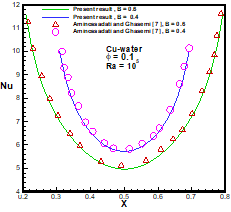(b)

Figure 2. (a) Comparison between our results and those of Oztop and Abu-nada  for the average Nusselt number Num at Ra=105 and f=0.10 (Cu–water nanofluid), (b) Comparison between our results and those of Aminossadati and Ghasemi  for the local Nusselt number Nu at Ra=105 and 0.10 (Cu–water nanofluid).

4. Results and Discussion

Steady state results presented in this paper are generated for different parameters: (104£Ra£106), solid volume fraction of nanoparticles (0£ϕ£0.10), dimensionless length of the heat source (0.50£B£1), and the type of nanofluids (Cu, Ag, Al2O3 and TiO2). The study deals with the effects of the Rayleigh number, solid volume fraction, type of nanofluids, and heat source length on the average Nusselt number ratio along the heat source and on the total entropy generation ratio.

4.1 Effects of Rayleigh number and solid volume fraction

Figure 3 represents Variation of average Nusselt number ratio (defined as : Num*=Num(0) /Num(f=0) ) with solid volume fraction f for various Rayleigh numbers Ra, at dimensionless heat source length B=0.5.With an increase of Rayleigh number, convective heat transfer and hence Nusselt number increases. It is found that the addition of nanoparticles has an effect on the average Nusselt number, indicating a better heat transfer. In addition, the effect of the nanoparticles is more significant at low Rayleigh number than at high Rayleigh number. For (Ra=104), the average Nusselt number ratio increases in a non-linear way with the increasing of Rayleigh number, because the heat transfer is associated to conduction and convection regime effect. For Ra>104, Num* increases linearly with the increase of Rayleigh number, this is justified by the higher buoyancy force effects, and the heat transfer inside the cavity is dominated by convection. In addition, the highest values for Nusselt number ratio are found at Ra = 106, where a stronger buoyant flow field appears in the enclosure. All figures show that the Nusselt numbers ratio Num*are starting from the same value. It is worth remarking that, the Rayleigh number values move away, according to the solid volume increasing.

Figure 4 shows the variation of total entropy generation ratio S* (defined as : S*=St(0) / St(f=0) ) with solid volume fraction f for various Rayleigh numbers Ra, at B=0.5.We show that the presence of nanoparticles has different effects on the production of entropy. The enhanced heat transfer due to the presence of nanoparticles increases the temperature

gradient and leads to a reduction of the production of entropy. The entropy generation due to heat transfer increases by increasing Ra and hence the buoyancy force. Nevertheless, the increase of Ra causes the reduction of entropy generation due to viscous effects. This fact, due to the increase of buoyancy effects which induces the flow intensity consequently causing reduction of viscosity. The effect of Ra in decreasing the total entropy generation, where viscous effects are dominant. The results show that the temperature gradient is the dominant factor in the entropy production, though; the effect of Ra is more considerable in nanofluid case than the base fluid in all the forms of entropy.

4.2 Effect of Nanofluids type

Figure 5 shows the variation of average Nusselt number ratio Num* with solid volume fraction f for various nanofluids, at B =0.5 and Ra=105. We show that the average Nusselt number ratio increases almost monotonically with increasing concentration for all nanofluids, and this increase is negligible for small values ​​of the Rayleigh number Ra (the natural convection mode). For Ra=105, we find the increase in the average Nusselt number ratio with the augmentation of the nanoparticles volume fraction, and this is justified by the increased heat transfer mode by convection. Also, we see that the average Nusselt ratio decreases as a function of the nanoparticle type (Ag, Cu, Al2O3, TiO2) and the lowest value of the average Nusselt ratio was obtained for TiO2 nanoparticles, this can be justified by the effect of the heat transfer mode by conduction and their low thermal conductivity compared to the other type of nanoparticles. However, the difference between the values ​​of the average Nusselt ratio of Ag and Cu is negligible; this is due to the thermal conductivity effect of the nanoparticle type as it has shown in table (1). In addition, we show that the average Nusselt number of the Ag and Cu nanoparticle are similar. We conclude that the highest value of the average Nusselt ratio is obtained for the type Ag nanoparticle.

Figure 6 represents the variation of total entropy generation ratio S* with solid volume fraction f for various nanofluids, at B =0.5 and Ra=105.We show that the total entropy generation vratio S* decreases depending on the types of nanoparticles (Ag, Cu, Al2O3 and TiO2), respectively. The total entropy generation ratio is always lower than the unit. Also, one can notice that, the increase in Rayleigh number corresponds to a reduction of total entropy generation ratio due to viscous effects; this is due to the effect of high heat transfer, and the higher temperature gradient. We conclude that the presence of nanoparticles plays a very important role in the entropy generation reduction. Where, these nanoparticles cause better heat transfer from the heater to the cold wall, which specifically generates smoother temperature distribution within the cavity and leads to the decrease in the total entropy generation.

4.3 Effects of the volume fraction for different heat source lengths

Figures 7a-b illustrate at Ra=105 the variation of average Nusselt number ratio Num* with solid volume fraction f for different values of B, and the total entropy generation ratio S* with solid volume fraction f for different B, respectively.We observe that in all cases (B = 0.5, 0.75, 1), the average Nusselt number ratio increases linearly with the increase of solid volume fraction(Fig.7a). It concluded that a high Rayleigh number is introduced by a strong buoyancy effect, and therefore, the transfer of heat inside the enclosure is dominated by convection. Note that the average Nusselt ratio increases with the decrease of B.

We notice that the entropy production rate decreased linearly with the increase of volume concentration, and indicates that increasing concentration causes the reduction of the total entropy ratio. We observe that the maximum value of the total entropy generation ratio is obtained for B=0.5.

Figure 8 shows the variation of total entropy generation ratio S*with solid volume fraction f for different viscosity and thermal conductivity models (see table 2), at Ra=105 and B=1. Entropy generation is due to both heat transfer and fluid friction irreversibility. We note that, the total entropy generation ratio decreases with the increase of the volume fraction for all models, because the increases of heat transfer. In the case of adding nanoparticles in the pure fluid, the nanoparticles have two effect on entropy generation production, the first one, with increasing the viscosity of fluid it conducts to the increase in viscous dissipation, so its outcome in increasing of entropy generation rate. The second one, augmenting the thermal conductivity enhances the heat transfer and it causes a reduction in the entropy generation, because of smaller temperature gradient existence. These two contrasting effects of nanoparticles presence lead to a decrease in entropy generation. One can observe that, all the figures have the same form, except the first type. Where, its entropy generation decreases linearly with the increase of the volume fraction for Rayleigh number 105. According to table (2), the conductivity value of this model is the lowest among the other models and heat transfer is low compared to the other models that produce a large entropy production. However, the curves of the other types are parabolic and they tend almost to zero because these models have high values of thermal conductivity. Therefore, the heat transfer is greater in comparison to the first model. The type 5 gives a lower total entropy generation S* in comparison to other models too.

## 3.pngFigure 3. Variation of average Nusselt number ratio Num* with solid volume fraction f for various Rayleigh numbers Ra, at dimensionless heat source length B=0.5.

## 4.pngFigure 4. Variation of total entropy generation ratio S* with solid volume fraction f for various Rayleigh numbers Ra, at B=0.5.

## 5.png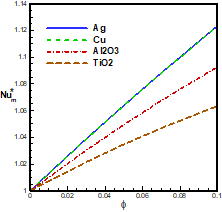Figure 5. Variation of average Nusselt number ratio Num* with solid volume fraction f for various nanofluids, at B =0.5 and Ra=105.

## 6.png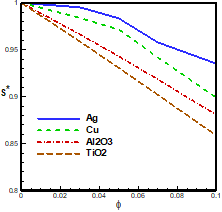Figure 6. Variation of total entropy generation ratio S* with solid volume fraction f for various nanofluids, at B =0.5 and Ra=105.

Table 2. Different models of the Thermal conductivity and dynamic viscosity

 Models Thermal conductivity Dynamic viscosity Model 1 Singh et al.  $k_{\mathrm{nf}}=\mathrm{k}_{\mathrm{f}}(1+4 \varphi)$ Brinkman  $\mu_{n f}=\frac{\mu_{f}}{(1-\varphi)^{2.5}}$ Model 2 Yu–Choi  $k_{\mathrm{nf}}=\frac{k_{p}+2 k_{f}+2\left(k_{p}-k_{f}\right)(1+\gamma)^{3} \varphi}{k_{p}+2 k_{f^{-}} 2\left(k_{p}-k_{f}\right)(1+\gamma)^{3} \varphi} k_{p}$ Brinkman  $\mu_{n f}=\frac{\mu_{f}}{(1-\varphi)^{25}}$ Model 3 Bruggeman  $\frac{k_{\mathrm{nf}}}{k_{\mathrm{f}}}=\frac{(3 \varphi-1) \frac{k_{\mathrm{p}}}{k_{\mathrm{f}}}+2 k_{f}+(3(1-\varphi)-1)+\sqrt{\Delta}}{4}$ $\Delta=[(3 \varphi-1)] \frac{\mathrm{k}_{\mathrm{p}}}{\mathrm{k}_{\mathrm{f}}}+[3(1-\varphi)-1]^{2}+8 \frac{k_{p}}{k_{f}}$ Maiga et al.  $\mu_{n f}=123\left(\phi^{2}+7.3 \phi+1\right)+\mu_{f}$ Model 4 Hamilton–Crosser  $k_{n f}=\frac{k_{p}+(n-1) k_{f}+(n-1) \phi\left(k_{p}-k_{f}\right)}{k_{p}+(n-1) k_{f}+\phi\left(k_{p}-k_{f}\right)}$ Maiga et al.  $\mu_{n f}=123\left(\varphi^{2}+7.3 \varphi+1\right)+\mu_{f}$ Model 5 Yu–Choi  $k_{\mathrm{nf}}=\frac{k_{p}+2 k_{f}+2\left(k_{p}-k_{f}\right)(1+\gamma)^{3} \varphi}{k_{p}+2 k_{f}-2\left(k_{p}-k_{f}\right)(1+\gamma)^{3} \varphi} k_{p}$ Maiga et al.  $\mu_{n f}=123\left(\varphi^{2}+7.3 \varphi+1\right)+\mu_{f}$

## 7a.png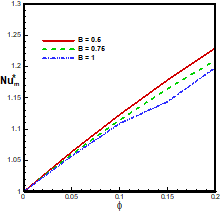(a)

## 7b.png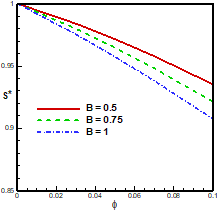(b)

Figure 7. (a) Variation of average Nusselt number ratio Num* with solid volume fraction f for different values of B, and (b) total entropy generation ratio S* with solid volume fraction f for different B.

5. Conclusion

This study has performed a numerical investigation about the natural convection heat transfer performance in a square cavity filled with water-based nanofluids. The governing equations were solved using the finite volume method. Results have shown that, for the considered Rayleigh numbers in this study (104-106) the average Nusselt number increases and the total entropy generation decreases with an increasing volume fraction of nanoparticles. The results also show that, the Cu-water nanofluid gives good performance of heat transfer and a weak total entropy production in the considered

nanofluids. In addition, the different type of viscosity and thermal conductivity have a big influence on the variation of total entropy generation.

## 8.pngFigure 8. Variation of total entropy  generation ratio S*with solid volume fraction f for different viscosity and thermal conductivity models (types), at Ra=105 and B=1.

Nomenclature
 B dimensionless heat source length CP specific heat, J. kg-1. K-1 g gravitational acceleration, m.s-2 k thermal conductivity, W.m-1. K-1 L cavity length, m Nu local Nusselt number along the heat source Num average Nusselt number U,V dimensionless velocity components u,v velocity components, m.s-1 x,y Cartesian coordinates, m X,Y dimensionless coordinats (x/L, y/L) P dimensionless pressure Ra Rayleigh number, (gβDTL3/ νfαf) Pr Prandtl number ((νf /αf) St dimensionless total entropy generation Greek symbols a thermal diffusivity, m2. s-1 b thermal expansion coefficient, K-1 f solid volume fraction Ɵ dimensionless temperature µ dynamic viscosity, kg. m-1.s-1 n kinematic viscosity, m2.s-1 r density, kg. m-3 Subscripts p nanoparticle f fluid (pure water) nf nanofluid C cold H hot
References

1. S.U.S. Choi, “Enhancing thermal conductivity of fluids with nanoparticles,” ASME Fluids Eng. Division, 231, 99–105, 1995.

2. Ho, C.J., Chen, M.W., and Li., Z.W, “Numerical simulation of natural convection of nanofluid in a square enclosure: effects due to uncertainties of viscosity and thermal conductivity,” International Journal of Heat and Mass Transfer, 51, 4506–4516, 2008. DOI: 10.1016/j.ijheatmasstransfer.2007.12.019.

3. Mahmoodi, M., and Sebdani, S.M., “Natural convection in a square cavity containing a nanofluid and an adiabatic square block at the center,” Superlattices and Microstructures, 52, 261–275, 2012. DOI: 10.1016/j.spmi.2012.05.007.

4. Jmai, R., Ben-Beya, B., and Lili, T., “Heat Transfer and Fluid Flow of Nanofluid-Filled Enclosure with Two Partially Heated Side Walls and Different Nanoparticles,” Superlattices and Microstructures, 53, 130–154, 2013. DOI: 10.1016/j.spmi.2012.10.003.

5. Tiwari, R.K., and Das, M.K., “Heat transfer augmentation in a two-sided lid-driven differentially heated square cavity utilizing nanofluids,” International Journal of Heat and Mass Transfer, 50, 2002–2018, 2007. DOI: 10.1016/j.ijheatmasstransfer.2006.09.034.

6. Ooi, E.H., and Popov, V., “Numerical study of influence of nanoparticle shape on the natural convection in cu-water nanofluid,” International Journal of Thermal Sciences, 65, 178-188, 2013. DOI: 10.1016/j.ijthermalsci.2012.10.020.

7. S.M. Aminossadati, B. Ghasemi, “Natural convection cooling of a localised heat source at the bottom of a nanofluid-filled enclosure,” European Journal of Mechanics B/Fluids, 28, 630–640, 2009. DOI: 10.1016/j.euromechflu.2009.05.006.

8. Aminossadati, S.M., and Ghasemi, B., “Enhanced natural convection in an isosceles triangular enclosure filled with a nanofluid,” Computers and Mathematics with Applications, 61, 1739–1753, 2011. DOI: 10.1016/j.camwa.2011.02.001.

9. K. Khanafer, K. Vafai, M. Lightstone, “Buoyancy- Driven Heat Transfer Enhancement in a Two- Dimensional Enclosure Utilizing Nanofluid-Filled,” International Journal of Heat and Mass Transfer, 46,3639–3653, 2003. DOI: 10.1016/S0017-9310(03)00156-X.

10. Salma Parvin, Rehena Nasrin, and M.A. Alim, “Heat transfer and entropy generation through nanofluid filled direct absorption solar collector,” International Journal of Heat and Mass Transfer, 71, 386–395, 2014. DOI: 10.1016/j.ijheatmasstransfer.2013.12.043.

11. Cheng, C.Y., “Free convection of non-newtonian nanofluids about a vertical truncated cone in a porous medium,” International Communications in Heat and Mass Transfer, 39, 1348–1353, 2012. DOI: 10.1016/j.icheatmasstransfer.2012.08.004.

12. Cheng, C.Y, “Free convection boundary layer flow over a horizontal cylinder of elliptic cross section in porous media saturated by a nanofluid,” International Communications in Heat and Mass Transfer, 39, 931–936, 2012. DOI: 10.1016/j.icheatmasstransfer.2012.05.014.

13. Cheng, C.Y., “Natural convection boundary layer flow over a truncated cone in a porous medium saturated by a nanofluid,” International Communications in Heat and Mass Transfer, 39, 231–235, 2012. DOI: 10.1016/j.icheatmasstransfer.2011.11.002.

14. Pawan K. Singh, K.B. Anoop, T.Sundararajan, Sarit K.Das, “Entropy generation due to flow and heat transfer in nanofluids,” International Journal of Heat and Mass Transfer, 53, 4757–4767, 2010. DOI: 10.1016/j.ijheatmasstransfer.2010.06.016.

15. Keblinski, S.R. Phillpot, S.U.S. Choi, J.A. Eastman, “Mechanism of heat flow in suspensions of nano-sized particles (nanofluids),” International Journal of Heat and Mass Transfer, 45, 855-863, 2002. DOI: 10.1016/S0017-9310(01)00175-2.

16. H. Saleh, R. Roslan, I. Hashim, “Natural Convection Heat Transfer in a Nanofluid-filled Trapezoidal Enclosure,” International Journal of Heat and Mass Transfer, 54, 194–201.2011. DOI: 10.1016/j.ijheatmasstransfer.2010.09.053.

17. Jou R.Y., and Tzeng S.C., “Numerical research of natural convective heat transfer enhancement filled with nanofluids in rectangular enclosures,” International Communications in Heat and Mass Transfer, 33,727–736, 2006. DOI: 10.1016/j.icheatmasstransfer.2006.02.016.

18. Oztop, H., Abu-Nada, E., Varol, Y., and Al-Salem, K., “Computational Analysis of Non-Isothermal Temperature Distribution on Natural Convection in Nanofluid Filled Enclosures,” Superlattices and Microstructures, 49, 453–467, 2011. DOI: 10.1016/j.spmi.2011.01.002.

19. T. Hayat, M. Hussain, A. Alsaedi, S.A. Shehzad, and G.Q. Chen, “Flow of power-law nanofluid over a stretching surface with newtonian heating,” Journal of Applied Fluid Mechanics, Vol.8, No.2, pp.273-280, 2015. DOI: 10.1371/journal.pone.0138855.

20. Ogut, E.B., “Natural convection of water-based nanofluids in an inclined enclosure with a heat source,” International Journal of Thermal Sciences, 48, 2063–2073, 2009. DOI: 10.1016/j.ijthermalsci.2009.03.014.

21. Abu-Nada, E., Oztop, F., “Effects of inclination angle on natural convection in enclosures filled with cu–water nanofluid,” International Journal of Heat and Fluid Flow, 30, 669–678, 2009. DOI: 10.1016/j.ijheatfluidflow.2009.02.001.

22. Mahmoodia, M., and Hashemi, S.M., “Numerical study of natural convection of a nanofluidin C-shaped enclosures,” International Journal of Thermal Sciences, 55, 76-89, 2012. DOI: 10.1016/j.ijthermalsci.2012.01.002.

23. Mina Shahi, Amir Houshang Mahmoudi, Abbas Honarbakhsh Raouf, “entropy generation due to natural convection cooling of a nanofluid,” International Communications in Heat and Mass Transfer, 38, 972–983, 2011. DOI: 10.1016/j.icheatmasstransfer.2011.04.008.

24. Patankar, S.V., Numerical Heat Transfer and Fluid Flow, McGraw-Hill, New York, 1980. DOI: 10.1002/cite.330530323.

25. H.C. Brinkman, “The viscosity of concentrated suspensions and solution,” J. Chem. Phys., 20, 571-581, 1952. DOI: 10.1063/1.1700493.

26. J. Maxwell, A Treatise on Electricity and Magnetism, second ed., Oxford University Press, Cambridge, UK, 1904. DOI: 10.1017/CBO9780511709333.

27. W. Yu, S.U.S. Choi, “The role of interfacial layers in the enhanced thermal of nanofluids: a Renovated Maxwell model,” J. Nanopart. Res., 5(1–2), 167–171, 2003. DOI: 10.1023/A:1024438603801.

28. D.A.G. Bruggeman, “Berechnung verschiedener physikalischer konstanten von heterogenen substanzen,” I. Dielektrizitatskonstanten und Leitfahigkeiten Dermischkorper aus Isotropen Substanzen, Ann. Phys. Leipzig 24, 636–679, 1935. DOI: 10.1002/andp.19354160705.

29. R.L. Hamilton, O.K. Crosser, “Thermal conductivity of heterogeneous two component systems,” I&EC Fundam., 1, 182–191, 1962. DOI: 10.1021/i160003a005.

30. S. Maiga, S.J. Palm, C.T. Nguyen, G. Roy, N. Galanis, “Heat transfer enhancement by using nanofluids in forced convection flows,” Int. J. Heat Fluid Flow, 26, 530–546, 2005. DOI: 10.1016/j.ijheatfluidflow.2005.02.004.